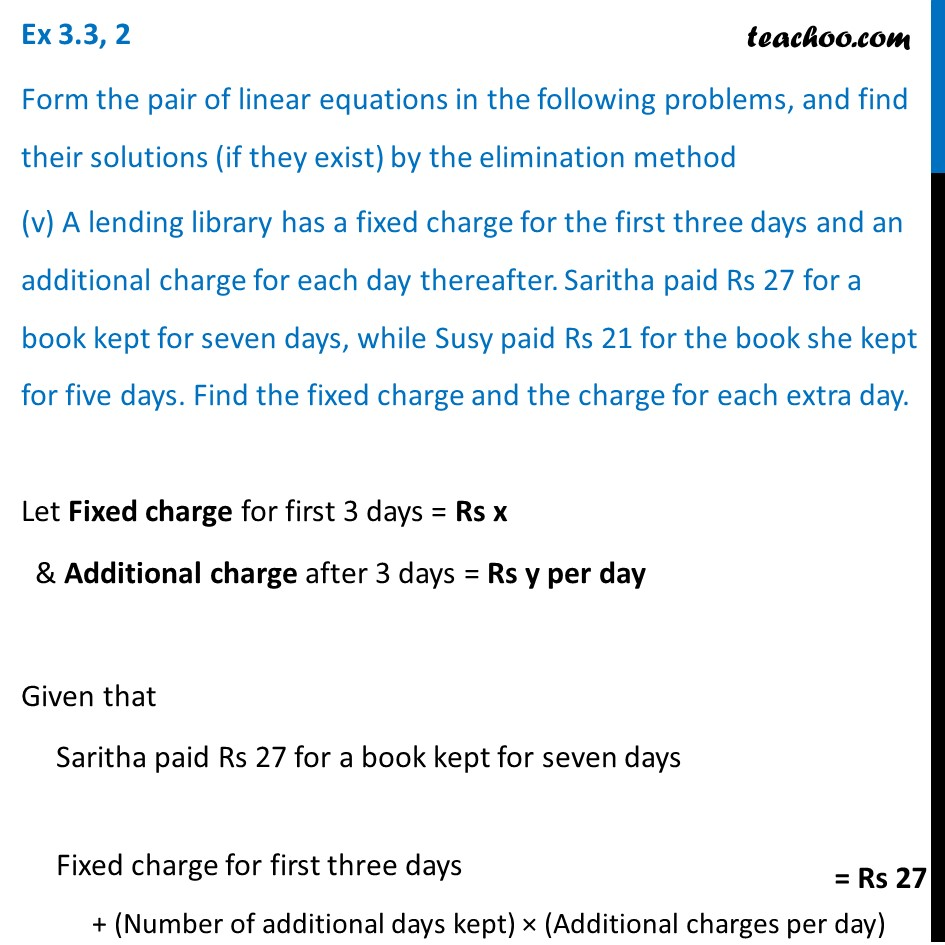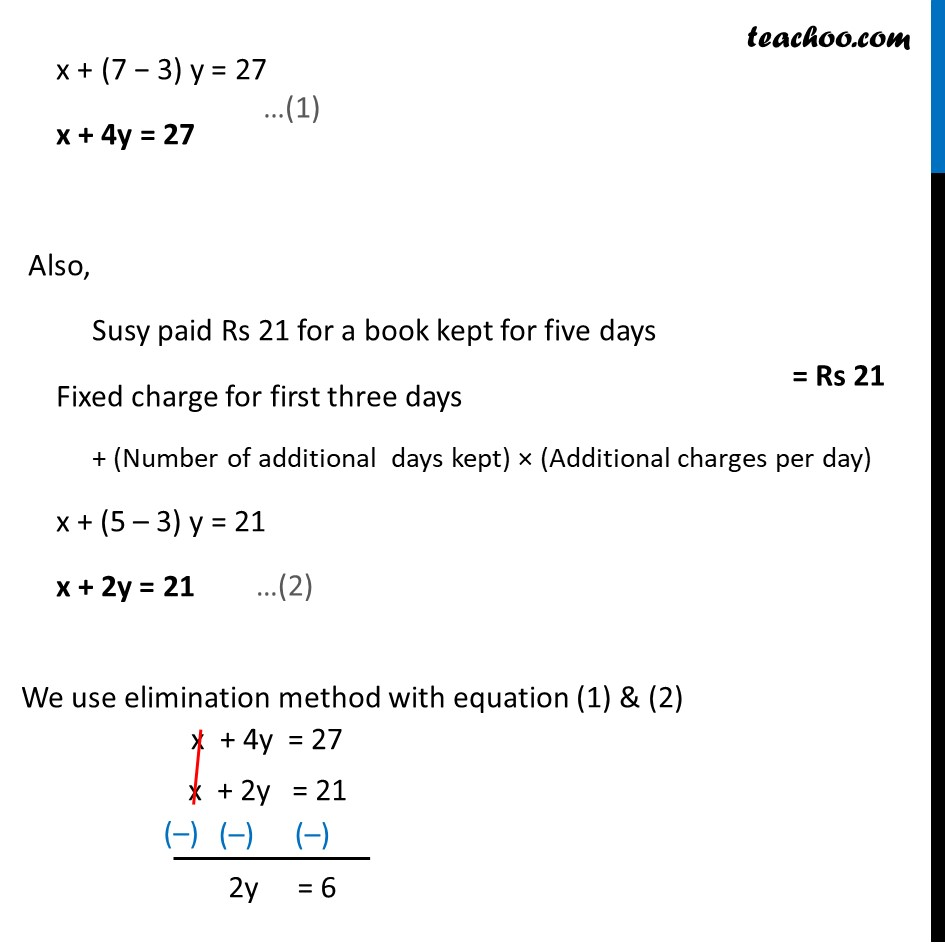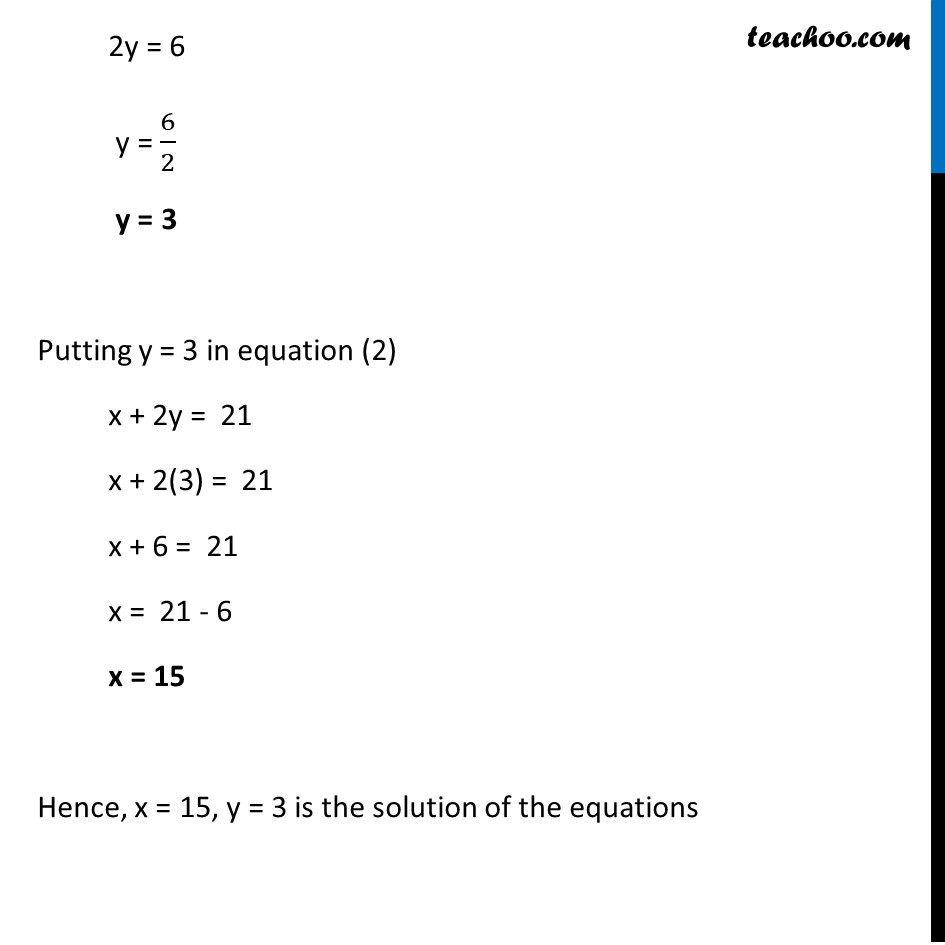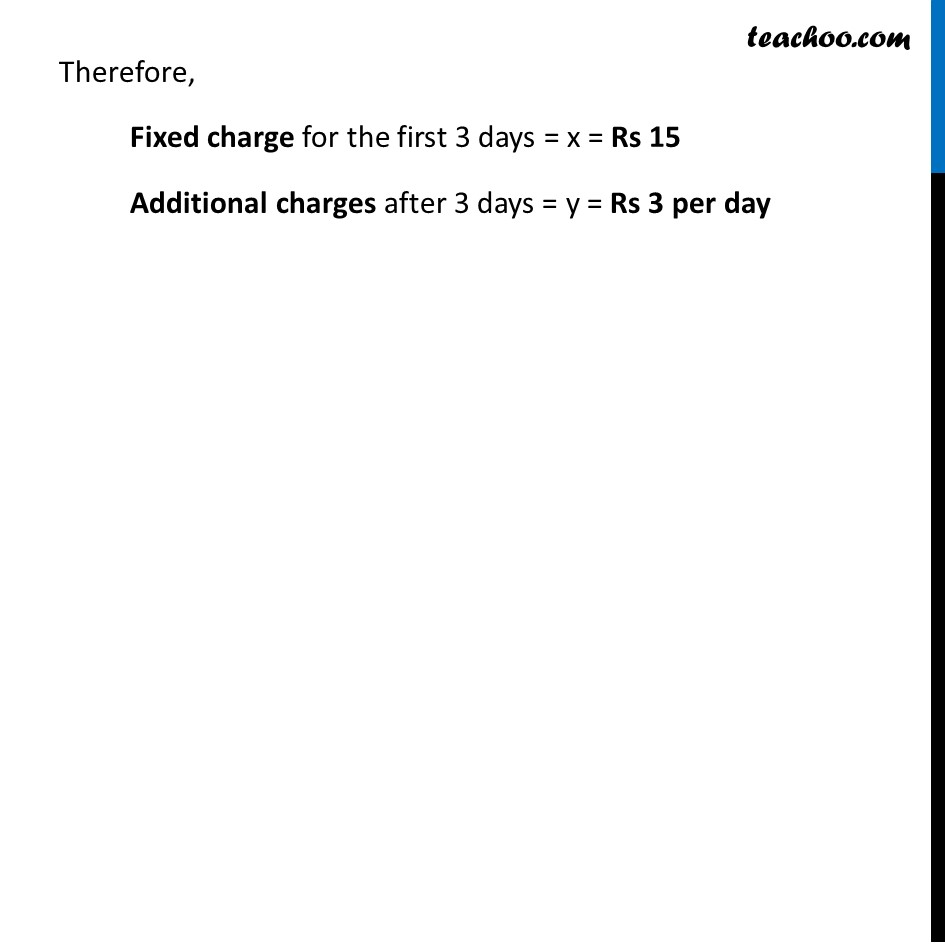Ex 3.3

Chapter 3 Class 10 Pair of Linear Equations in Two Variables
Serial order wiseLearn in your speed, with individual attention - Teachoo Maths 1-on-1 Class

### Transcript

Ex 3.3, 2 Form the pair of linear equations in the following problems, and find their solutions (if they exist) by the elimination method (v) A lending library has a fixed charge for the first three days and an additional charge for each day thereafter. Saritha paid Rs 27 for a book kept for seven days, while Susy paid Rs 21 for the book she kept for five days. Find the fixed charge and the charge for each extra day. Let Fixed charge for first 3 days = Rs x & Additional charge after 3 days = Rs y per day Given that Saritha paid Rs 27 for a book kept for seven days Fixed charge for first three days + (Number of additional days kept) × (Additional charges per day) "x + (7 − 3) y = 27" "x + 4y = 27" Also, Susy paid Rs 21 for a book kept for five days Fixed charge for first three days + (Number of additional days kept) × (Additional charges per day) x + (5 – 3) y = 21 x + 2y = 21 We use elimination method with equation (1) & (2) 2y = 6 y = 6/2 y = 3 Putting y = 3 in equation (2) x + 2y = 21 x + 2(3) = 21 x + 6 = 21 x = 21 - 6 x = 15 Hence, x = 15, y = 3 is the solution of the equations Therefore, Fixed charge for the first 3 days = x = Rs 15 Additional charges after 3 days = y = Rs 3 per day